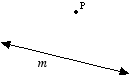index: click on a letter A B C D E F G H I J K L M N O P Q R S T U V W X Y Z A to Z index index: subject areas numbers & symbols sets, logic, proofs geometry algebra trigonometry advanced algebra & pre-calculus calculus advanced topics probability & statistics real world applications multimedia entrieswww.mathwords.com about mathwords website feedback

 Hyperbolic Geometry A non-Euclidean geometry with the following property: Given a line m and a point P not on m, there are infinitely many lines passing through P which are parallel to m. Hyperbolic geometry may be thought of as plane geometry on a surface shaped like the bell of a trumpet.See also Elliptic geometry